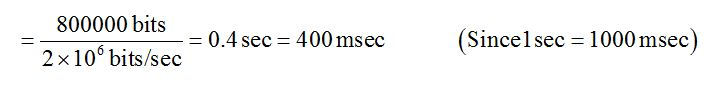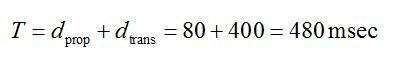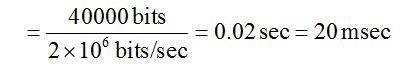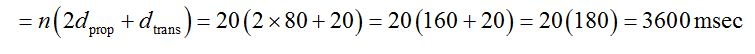SHARE
HELP

To start with, Sr2Jr’s first step is to reduce the expenses related to education. To achieve this goal Sr2Jr organized the textbook’s question and answers. Sr2Jr is community based and need your support to fill the question and answers. The question and answers posted will be available free of cost to all.Authors:
James F. Kurose, Keith W. Ross
Chapter:
Computer Networks And The Internet
Exercise:
Problems
Question:28 | ISBN:9780132856201 | Edition: 6

###Question

Refer again to problem P25.

a. How long does it take to send the file, assuming it is sent continuously?

b. Suppose now the file is broken up into 20 packets with each packet containing 40,000 bits. Suppose that each packet is acknowledged by the receiver and the transmission time of an acknowledgment packet is negligible. Finally, assume that the sender cannot send a packet until the preceding one is acknowledged. How long does it take to send the file?

c. Compare the results from (a) and (b).

###Answer

As per problem 25, two hosts (A and B) are connected using direct link.

The distance between these two hosts A and B = 20,000km

= 20000x10^3 m

=2x10^7 m

Transmission rate (speed) of the direct link between A and B(R) = 2Mbps

= 2 x 10^6 bps

Propagation speed of the link between A and B(S) = 2.5x10^8 meters/sec

a)

Here, length of the file is transmitted continuously and it is represented as L.

The length of the file (L) = 800,000bits

Calculating the propagation delay = distance/speed

= 2x10^7 / 2.5 x 10^8  = 0.08sec = 80msec

Calculate the transmission delay =The time required for transmitting the file continuously =b)

The length of the file (L) = 800,000bits

The file is divided into 20 packets. So, the length of each packet =40,000bits

Calculating the propagation delay = distance/speed

= 2x10^7 / 2.5 x 10^8    = 0.08sec  = 80msec

Calculate the transmission delay =Calculate the total time required to transmit n packets:c)

The time taken to transfer the file continuously from the host A to B is 480 msec as per part(a).

The time taken to transfer the file by divided into multiple packets from the host A to B is 3600 msec as per part(b).

Hence, transmitting the file continuously is more efficient than transmitting the file as multiple packets.

### Discussions

Post the discussion to improve the above solution.